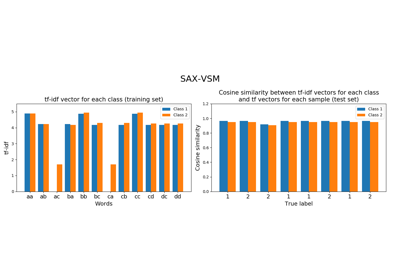# `pyts.classification`.SAXVSM¶

class `pyts.classification.``SAXVSM`(n_bins=4, strategy='quantile', window_size=4, window_step=1, numerosity_reduction=True, use_idf=True, smooth_idf=False, sublinear_tf=True, alphabet=None)[source]

Classifier based on SAX-VSM representation and tf-idf statistics.

Time series are first transformed into bag of words using Symbolic Aggregate approXimation (SAX) algorithm followed by a bag-of-words model. Then the classes are transformed into a Vector Space Model (VSM) using term frequencies (tf) and inverse document frequencies (idf).

Parameters: n_bins : int (default = 4) The number of bins to produce. It must be between 2 and `min(n_timestamps, 26)`. strategy : ‘uniform’, ‘quantile’ or ‘normal’ (default = ‘quantile’) Strategy used to define the widths of the bins: ‘uniform’: All bins in each sample have identical widths ‘quantile’: All bins in each sample have the same number of points ‘normal’: Bin edges are quantiles from a standard normal distribution window_size : int or float (default = 4) Size of the sliding window (i.e. the size of each word). If float, it represents the percentage of the size of each time series and must be between 0 and 1. The window size will be computed as `ceil(window_size * n_timestamps)`. window_step : int or float (default = 1) Step of the sliding window. If float, it represents the percentage of the size of each time series and must be between 0 and 1. The window step will be computed as `ceil(window_step * n_timestamps)`. numerosity_reduction : bool (default = True) If True, delete sample-wise all but one occurence of back to back identical occurences of the same words. use_idf : bool (default = True) Enable inverse-document-frequency reweighting. smooth_idf : bool (default = False) Smooth idf weights by adding one to document frequencies, as if an extra document was seen containing every term in the collection exactly once. Prevents zero divisions. sublinear_tf : bool (default = True) Apply sublinear tf scaling, i.e. replace tf with 1 + log(tf). alphabet : None or array-like, shape = (n_bins,) Alphabet to use. If None, the first n_bins letters of the Latin alphabet are used.

References

 [R329e95927982-1] P. Senin, and S. Malinchik, “SAX-VSM: Interpretable Time Series Classification Using SAX and Vector Space Model”. International Conference on Data Mining, 13, 1175-1180 (2013).

Examples

```>>> from pyts.classification import SAXVSM
>>> X_train, X_test, y_train, y_test = load_gunpoint(return_X_y=True)
>>> clf = SAXVSM(window_size=34, sublinear_tf=False, use_idf=False)
>>> clf.fit(X_train, y_train) # doctest: +ELLIPSIS
SAXVSM(...)
>>> clf.score(X_test, y_test)
0.76
```
Attributes: classes_ : array, shape = (n_classes,) An array of class labels known to the classifier. idf_ : array, shape = (n_features,) , or None The learned idf vector (global term weights) when `use_idf=True`, None otherwise. tfidf_ : array, shape = (n_classes, n_words) Term-document matrix. vocabulary_ : dict A mapping of feature indices to terms.

Methods

 `__init__`(self[, n_bins, strategy, …]) Initialize self. `decision_function`(self, X) Evaluate the cosine similarity between document-term matrix and X. `fit`(self, X, y) Fit the model according to the given training data. `get_params`(self[, deep]) Get parameters for this estimator. `predict`(self, X) Predict the class labels for the provided data. `score`(self, X, y[, sample_weight]) Return the mean accuracy on the given test data and labels. `set_params`(self, \*\*params) Set the parameters of this estimator.
`__init__`(self, n_bins=4, strategy='quantile', window_size=4, window_step=1, numerosity_reduction=True, use_idf=True, smooth_idf=False, sublinear_tf=True, alphabet=None)[source]

Initialize self. See help(type(self)) for accurate signature.

`decision_function`(self, X)[source]

Evaluate the cosine similarity between document-term matrix and X.

Parameters: X : array-like, shape (n_samples, n_timestamps) Test samples. X : array-like, shape (n_samples, n_classes) osine similarity between the document-term matrix and X.
`fit`(self, X, y)[source]

Fit the model according to the given training data.

Parameters: X : array-like, shape = (n_samples, n_timestamps) Training vector. y : array-like, shape = (n_samples,) Class labels for each data sample. self : object
`get_params`(self, deep=True)

Get parameters for this estimator.

Parameters: deep : bool, default=True If True, will return the parameters for this estimator and contained subobjects that are estimators. params : mapping of string to any Parameter names mapped to their values.
`predict`(self, X)[source]

Predict the class labels for the provided data.

Parameters: X : array-like, shape = (n_samples, n_timestamps) Test samples. y_pred : array-like, shape = (n_samples,) Class labels for each data sample.
`score`(self, X, y, sample_weight=None)

Return the mean accuracy on the given test data and labels.

In multi-label classification, this is the subset accuracy which is a harsh metric since you require for each sample that each label set be correctly predicted.

Parameters: X : array-like of shape (n_samples, n_features) Test samples. y : array-like of shape (n_samples,) or (n_samples, n_outputs) True labels for X. sample_weight : array-like of shape (n_samples,), default=None Sample weights. score : float Mean accuracy of self.predict(X) wrt. y.
`set_params`(self, **params)

Set the parameters of this estimator.

The method works on simple estimators as well as on nested objects (such as pipelines). The latter have parameters of the form `<component>__<parameter>` so that it’s possible to update each component of a nested object.

Parameters: **params : dict Estimator parameters. self : object Estimator instance.

## Examples using `pyts.classification.SAXVSM`¶Symbolic Aggregate approXimation in Vector Space Model (SAX-VSM)